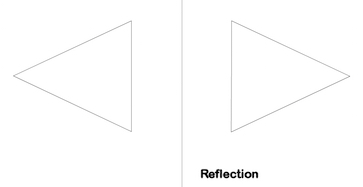• Turn off Ads!
•Create A Profile

# Page 3 Mathematics Study Guide for the PRAXIS® test

### Geometry

Geometry is the study of points, lines, angles, shapes, and the nature of space. Principles of geometry are used to derive properties to compare and construct two and three dimensional figures.

#### Plane Figures

Plane figures are shapes drawn in two dimensions, such as on a sheet of paper. In geometry the regular plane figures such as rectangles, triangles, and circles have specific definitions and properties. These definitions can be used to draw or construct each figure. Some properties, such as perimeter and area, can be used to compare the figures. The example figure shows a rectangle with length 5 and width 3 and a circle with radius 4. The area of the rectangle is 15 and the area of the circle is approximately 50.Congruency

Two figures are congruent when they have the same size and shape. If superimposed they would fit on each other exactly. The measures of corresponding angles and sides are the same in both figures. The example shows a pair of congruent triangles.Similarity

Similar figures are the same shape but not the same size. If the smaller figure is expanded by the correct amount it could be superimposed on the other figure. The measures of corresponding angles are the same, but the measures of the sides are proportionately larger or smaller. The example shows a pair of similar triangles.Transformation

A transformation can be thought of as a way to move or change a geometric figure. There are four types of transformations: translations, rotations, reflections, and dilations. An example of each transformation is shown in these figures:Angles

An angle is formed by two rays, called the sides of the angle, originating from a common point called the vertex. Angles are typically measured in degrees of arc. Angles are classified by their size in degrees. Acute angles have a measure of less than 90 degrees. Right angles have a measure of 90 degrees. Obtuse angles have a measure greater than 90 and less than 180 degrees. Straight angles have a measure of 180 degrees and are formed by a straight line.

Right Triangles

Right triangles contain one right angle. An important property of right triangles is expressed by the Pythagorean theorem. The theorem describes the relationship between the length of the side opposite the right angle, or hypotenuse, represented as the variable c, and the lengths of the other two sides, represented by the variables a and b. $a^{2} + b^{2} = c^{2}$.

Dimensions and Perimeter

Geometric figures are often defined by dimensions such as length, width, and height. The perimeter of a figure is the distance around the edge of the figure and is calculated from the measurements of the dimensions. The perimeter of a circle is called the circumference.Area

Area is a measure of the space contained within a figure. It is measured in square units. For example if the dimensions of the figure are given in inches, the area is measured in square inches. Some examples are shown in the accompanying figure.Circles

A circle is defined as a set of points equidistant from a point called the center. The radius of a circle is the measure of a line segment from the center to the edge of the circle. The diameter of a circle is a line segment that passes through the center and has its endpoints on the circle. The length of the diameter is twice the length of the radius. The area of a circle is given by the formula $A = \pi r^{2}$ and the circumference is given by the formula $C = 2 \pi r$, where r is the radius of the circle.#### 3-D Figures

3-D figures are solid shapes such as spheres, cylinders, and cubes. Their basic characteristics include length, height, depth, and radius. Other characteristics such as surface area and volume can be calculated from the basic characteristics.

Surface Area

The surface area of a 3-D figure is the total area of all of its faces or outer surface. It can be thought of as the area covering it if each surface was joined on a flat surface.

The surface area of shapes such as the rectangular cuboid can be calculated by adding the areas of all of the faces on the shape.The surface area of a sphere is given by the formula $S = 4\pi r^{2}$.The surface area of a cylinder is calculated by finding the surface area of the circles on the top and bottom, then adding it to the area of the rectangle that curves to form the sides of the cylinder.Volume

The volume of a 3-D figure is the total amount of space contained by the figure. If the figure could be filled with water, the volume would be the amount of water it holds. Volume is measured in cubic units. For example, if the dimensions of the figure are given in inches, the volume is measured in cubic inches. Some formulas for the volume of 3-D figures are:

Cube with side s: $V = s^{3}$

Rectangular prism with sides a, b, c: $V = abc$

Cylinder with base radius r and height h: $V = \pi r^{2}h$

Sphere with radius r: $V = \frac{4}{3}\pi r^{3}$

Modeling

Geometric shapes and concepts can be used to describe objects, describe density using area or volume, and solve design problems with cost or size constraints. An example could be to use a given length of fencing (the perimeter) to fence a garden with the most area.